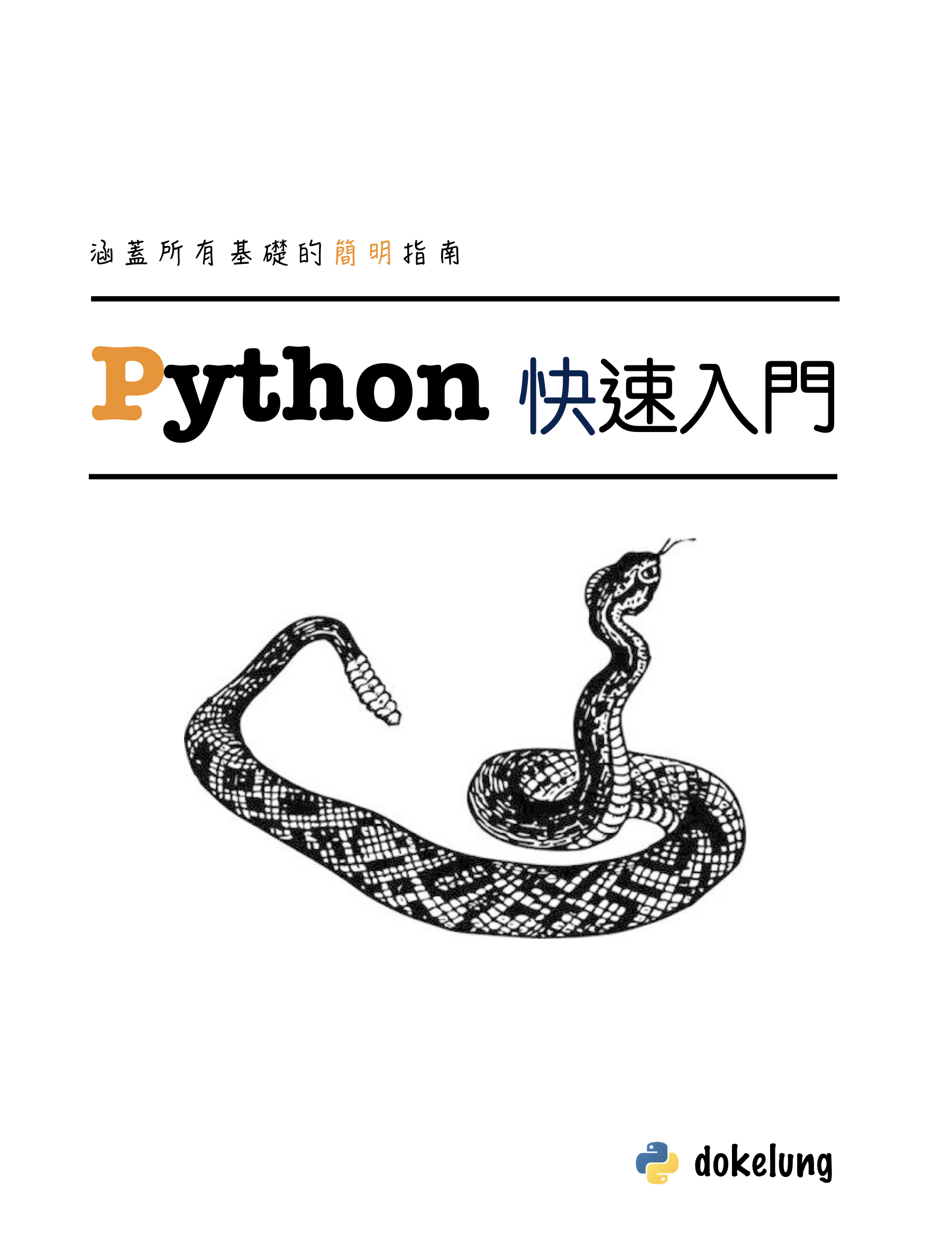# Don't Reinvent Sandwich: Decorator## 從檔案的開關談起

Python 的檔案操作一直都是很平易近人的，使用內建的 `open` 函數來開啟檔案並且獲得回傳的 檔案物件 (file object)，最後透過檔案物件的 `close` 方法關閉檔案，大概像是這樣子：

```fp = open(file_name, 'w')

# do somethings here

fp.close() # 這行很容易被遺忘
```

```fp = open(file_name, 'w')
try:
# do somethings here
finally:
fp.close()
```

```with open(file_name, 'w') as fp:
# do somethings here
```

## 裝飾器 (decorator)

### 裝飾器的用法

```import time

def snooze(s):
"""等待s秒"""
time.sleep(s)

def sum_of_square(numbers):
"""計算平方和"""
return sum([n**2 for n in numbers])
```

```import time

def snooze(s):
"""等待s秒"""
t0 = time.time()
time.sleep(s)
elapsed = time.time()-t0
print('Run snooze({}): {}'.format(s, elapsed))

def sum_of_square(numbers):
"""計算平方和"""
t0 = time.time()
result = sum([n**2 for n in numbers])
elapsed = time.time()-t0
print('Run sum_of_square({}): {}'.format(numbers, elapsed))
return result
```

```import time

@clock
def snooze(s):
"""等待s秒"""
time.sleep(s)

@clock
def sum_of_square(numbers):
"""計算平方和"""
return sum([n**2 for n in numbers])
```

```import time

def snooze(s):
"""等待s秒"""
time.sleep(s)

snooze = clock(snooze)

def sum_of_square(numbers):
"""計算平方和"""
return sum([n**2 for n in numbers])

sum_of_square = clock(sum_of_square)
```

```裝飾器是一個函數，他能接收一個函數作為參數，並且回傳一個修飾過的函數
```

```# 通常不會為修飾過的函數另取名稱
clock_snooze = clock(snooze)
clock_sum_of_square = clock(sum_of_square)

# 通常會使用原本的名稱
snooze = clock(snooze)
sum_of_square = clock(sum_of_square)
```

### 裝飾器的寫法

```def clock(func):
# ...
```

```def clock(func):
def new_func(...):
"""裝飾過的函數"""
# ...
return new_func
```

```def clock(func):
def new_func(...):
"""裝飾過的函數"""
result = func(...)
return result
return new_func
```

```def clock(func):
# 使用星號讓新的函數能夠接受任意參數，換句話說能讓裝飾器裝飾任意函數
def new_func(*args, **kwargs):
"""裝飾過的函數"""
result = func(*args, **kwargs) # 呼叫原本的函數時也使用星號來進行參數拆解
return result
return new_func
```

```def clock(func):
def new_func(*args, **kwargs):
"""裝飾過的函數"""
t0 = time.time()
result = func(*args, **kwargs)
elapsed = time.time() - t0

# func 的 __name__ 屬性儲存著函數的名字
name = func.__name__

# 將呼叫時的參數值轉為可印出的字串
arg_strs = [repr(arg) for arg in args]
kwarg_strs = ['{}={}'.format(key, value) for key, value in kwargs.items()]
argstr = ','.join(arg_strs + kwarg_strs)

print('Run {}({}): {}'.format(name, argstr, elapsed))
return result
return new_func
```

### 使用 wraps 來改善裝飾器

```# 這裡的 snooze 是用 clock 裝飾過的版本
>>> snooze.__name__
'new_func'
>>> snooze.__doc__
```

```>>> help(snooze)

Help on function new_func in module __main__:

new_func(*args, **kwargs)
```

```from functools import wraps

def clock(func):
# wraps 會將 func 的屬性抄到 new_func 裡讓 new_func 看起來跟 func 一模一樣
@wraps(func)
def new_func(*args, **kwargs):
"""裝飾過的函數"""
t0 = time.time()
result = func(*args, **kwargs)
elapsed = time.time() - t0
name = func.__name__
arg_strs = [repr(arg) for arg in args]
kwarg_strs = ['{}={}'.format(key, value) for key, value in kwargs.items()]
argstr = ','.join(arg_strs + kwarg_strs)
print('Run {}({}): {}'.format(name, argstr, elapsed))
return result
return new_func
```

### 帶有參數的裝飾器

```@tag('div')
@tag('p')
def content():
print('content line')
```

```>>> content()
<div>
<p>
content line
</p>
</div>
```

`tag` 這個裝飾器可以在原函數的輸出前後印出指定的 html tag 頭尾，我們要來研究兩個點：

1. 如何寫出帶有參數的裝飾器
2. 裝飾器的堆疊

```def tag(func):
@wraps(func)
def new_func():
# do somethings before
func()
# do somethings after
return new_func
```

```def tag(func):
@wraps(func)
def new_func():
print('<{}>'.format(TAG_NAME))
func()
print('</{}>'.format(TAG_NAME))
return new_func
```

```def tag(func, name):
@wraps(func)
def new_func():
print('<{}>'.format(name))
func()
print('</{}>'.format(name))
return new_func
```

```content = tag(content, 'p')
```

```def tag(name):
# 這個才是真正的裝飾器，為了把 tag 讓給更上一層的函數，這裡我們使用 deco 這個名字
def deco(func):
@wraps
def new_func():
print('<{}>'.format(name))
func()
print('</{}>'.format(name))
return new_func
return deco
```

```ptag = tag('p') # ptag 才是真正的裝飾器
content = ptag(content)

# 或是使用 @ 的語法寫出來
@ptag
def content():
print('content line')

# 或直接在 @ 的那一行呼叫 tag 製造真正的裝飾器
@tag('p') # 這一行相當於 @ptag，也就是說 tag('p') 才是直接作用的裝飾器，tag 只是製造裝飾器的函數
def content():
print('content line')
```

### 裝飾器的堆疊

```@tag('div')
@tag('p')
def content():
print('content line')
```

```divtag = tag('div')
ptag = tag('p')

content = content(divtag(ptag(content)))
```

`content` 會先被 `ptag` 也就是 `tag('p')` 裝飾，接著才被 `divtag` 也就是 `tag('div')` 裝飾。其實只要我們將裝飾器的甜頭語法還原回去，便很容易理解裝飾器的原理和行為。

```@divtag
@ptag
def content():
print('content line')
```

### 裝飾器的範例

• function log
• top function exception
• click_
• cache_json(mock function by modified file)

### 常用的內建裝飾器

• `property`
• `classmethod`
• `staticmethod`

• `functools.wraps`
• `functools.lru_cache`
• `functools.singeldispatch`

### functools.lru_cache

```@clock # 使用前面我們自行定義的 clcok 裝飾器
def fibonacci(n):
"""求取費氏數列的第n項"""
if n < 2:
return n
return fibonacci(n-1) + fibonacci(n-2)
```

```>>> fibonacci(6)
Run fibonacci(1): 9.5367431640625e-07
Run fibonacci(0): 1.9073486328125e-06
Run fibonacci(2): 0.0001251697540283203
Run fibonacci(1): 9.5367431640625e-07
Run fibonacci(3): 0.0001609325408935547
Run fibonacci(1): 9.5367431640625e-07
Run fibonacci(0): 0.0
Run fibonacci(2): 3.695487976074219e-05
Run fibonacci(4): 0.00023412704467773438
Run fibonacci(1): 1.1920928955078125e-06
Run fibonacci(0): 0.0
Run fibonacci(2): 3.409385681152344e-05
Run fibonacci(1): 1.1920928955078125e-06
Run fibonacci(3): 7.200241088867188e-05
Run fibonacci(5): 0.00033593177795410156
Run fibonacci(1): 0.0
Run fibonacci(0): 1.1920928955078125e-06
Run fibonacci(2): 2.8133392333984375e-05
Run fibonacci(1): 9.5367431640625e-07
Run fibonacci(3): 6.389617919921875e-05
Run fibonacci(1): 0.0
Run fibonacci(0): 9.5367431640625e-07
Run fibonacci(2): 3.504753112792969e-05
Run fibonacci(4): 0.00013399124145507812
Run fibonacci(6): 0.0005078315734863281
8
```

```dict = {}

@clock # 使用前面我們自行定義的 clcok 裝飾器
def fibonacci(n):
"""求取費氏數列的第n項"""
if n in dict:
pass
elif n < 2:
dict[n] = n
else:
dict[n] = fibonacci(n-1) + fibonacci(n-2)
return dict[n]
```

```from functools import lru_cache

@lru_cache()
@clock # 使用前面我們自行定義的 clcok 裝飾器
def fibonacci(n):
"""求取費氏數列的第n項"""
if n < 2:
return n
return fibonacci(n-1) + fibonacci(n-2)
```

```>>> fibonacci(6)
Run fibonacci(1): 1.1920928955078125e-06
Run fibonacci(0): 2.1457672119140625e-06
Run fibonacci(2): 0.00012111663818359375
Run fibonacci(3): 0.00014090538024902344
Run fibonacci(4): 0.0001609325408935547
Run fibonacci(5): 0.0001819133758544922
Run fibonacci(6): 0.0002009868621826172
8
```

`lru_cache` 的原理很簡單，他利用 字典(dictionary) 來作為緩存區，每一次的函數呼叫，都會將該次呼叫的引數當作字典的 鍵(key)，將其回傳值當作字典的 值(value)，將此鍵值對存入字典中以便下次查詢。

`lru_cache` 有兩個可選的參數，一個是 `maxsize`，用以指定緩存區的大小，代表著有多少呼叫的結果會被儲存，一旦緩存被填滿，較舊的結果將會被丟棄。

### 用裝飾器來註冊函數

```def deco(func):
@wraps(func)
def new_function(*args, **kwargs):
# 裝飾點1: 在原函數呼叫之前做些什麼...
result = func(*args, **kwargs)
# 裝飾點2: 在原函數呼叫之後做些什麼...
return result
return new_function
```

```def deco(func):
# 裝飾點3: 在裝飾的當下註冊函數 func ...
@wraps(func)
def new_function(*args, **kwargs):
# 裝飾點1: 在原函數呼叫之前做些什麼 ...
result = func(*args, **kwargs)
# 裝飾點2: 在原函數呼叫之後做些什麼 ...
return result
return new_function
```

```from functools import wraps

url_mapping = {}

def myroute(url):
def deco(view_func):
url_mapping[url] = view_func # 註冊 url 及其對應的 view function
return view_func # 把原函數當成修飾過的函數即可，這次的重點在於註冊，而非改變原函數行為
return deco
```

```@myroute('/')
def index():
return 'Index Page'

@myroute('/hello')
def hello():
return 'Hello, World'
```

```>>> url_mapping
{'/': <function __main__.index>, '/hello': <function __main__.hello>}
```

## 參考資料

### dokelungHi, my name is Ko-Lung Yuan!

book

### It's djangoA book about python web framework Django

book

### Python 快速入門